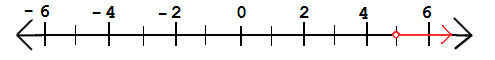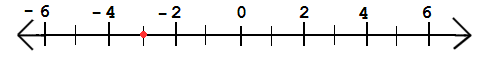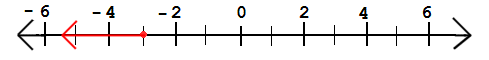# Simple Inequalities

 Our Pre-Algebra tutorial software program contains over 60 topic areas. One of them is Simple Inequalities, and this page summarizes the main ideas of this topic. This page is intended for review, and is not a substitute for the interactive, self-paced tutorials of the MathTutor pre-algebra software program.

General Explanation

An INEQUALITY is a statement which shows that two quantities are not equal. If two quantities are not equal, then one is greater than the other.

The symbol for equality is "=".

The symbols for inequality are:
```">" for "greater than"
"<" for "less than"
```
The following expression states that x is greater than 6:
` x > 6 `
The following states that x is greater than or equal to 6:
` x ≥ 6`
Similarly, the following states that x is less than or equal to 6:
` x ≤ 6`
On the number line, x > 6 represents all the numbers to the right of 6, while x ≥ 6 means 6 and all the numbers to the right of 6. Similarly, x < 6 represents all the numbers to the left of 6, while x ≤ 6 represents 6 and all the numbers to the left of 6.

Sample Problem 1

Write an inequality to represent the numbers shown by the red arrow in the following graph:
``

Solution

The arrow contains points to the right of the number 5, but it does not include 5 because there is an open circle over the number. So the arrow represents all the numbers greater than 5.

This can be represented by the following inequality:
` x > 5`

Sample Problem 2

Draw a graph for the inequality:
` x ≤ -3`

Solution

We see that the inequality contains a "less than or equal to" sign, so we know the graph will include the point -3. We fill in a circle at this point:
``
Since x is LESS than or equal to -3, we draw the arrow to the left:
``
The red arrow represents the inequality x ≤ -3.